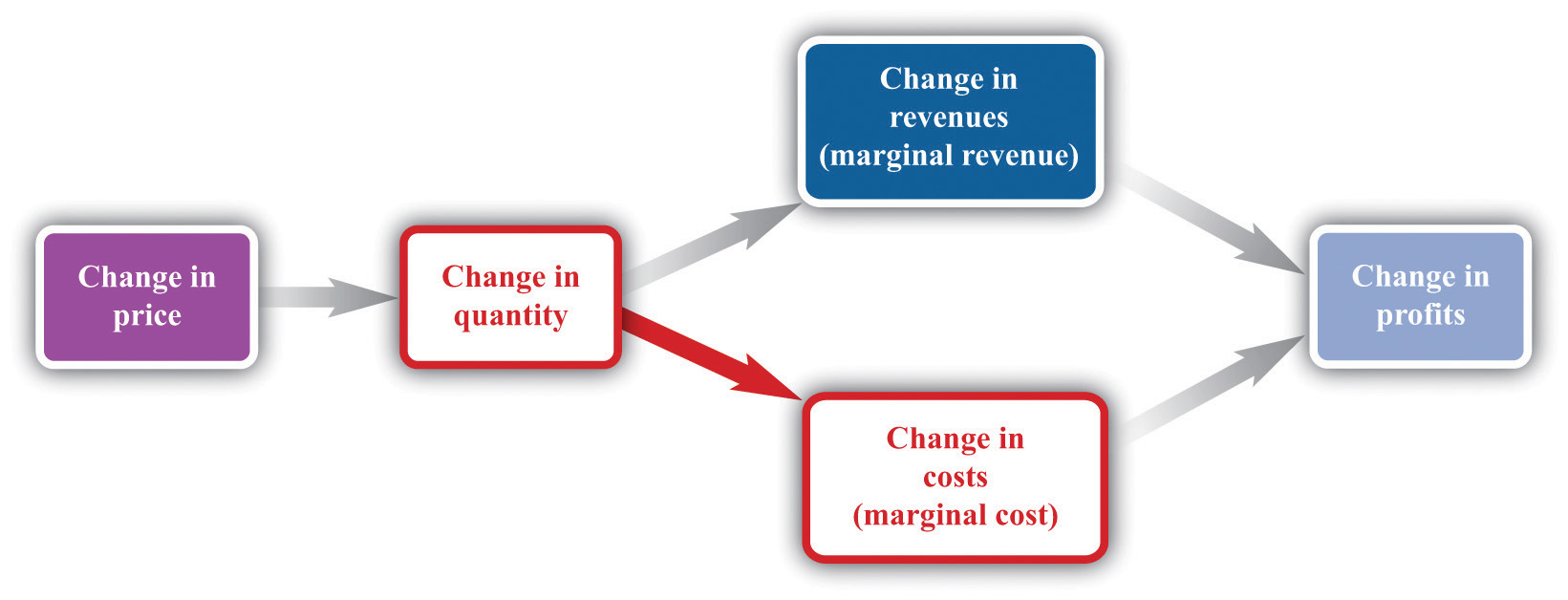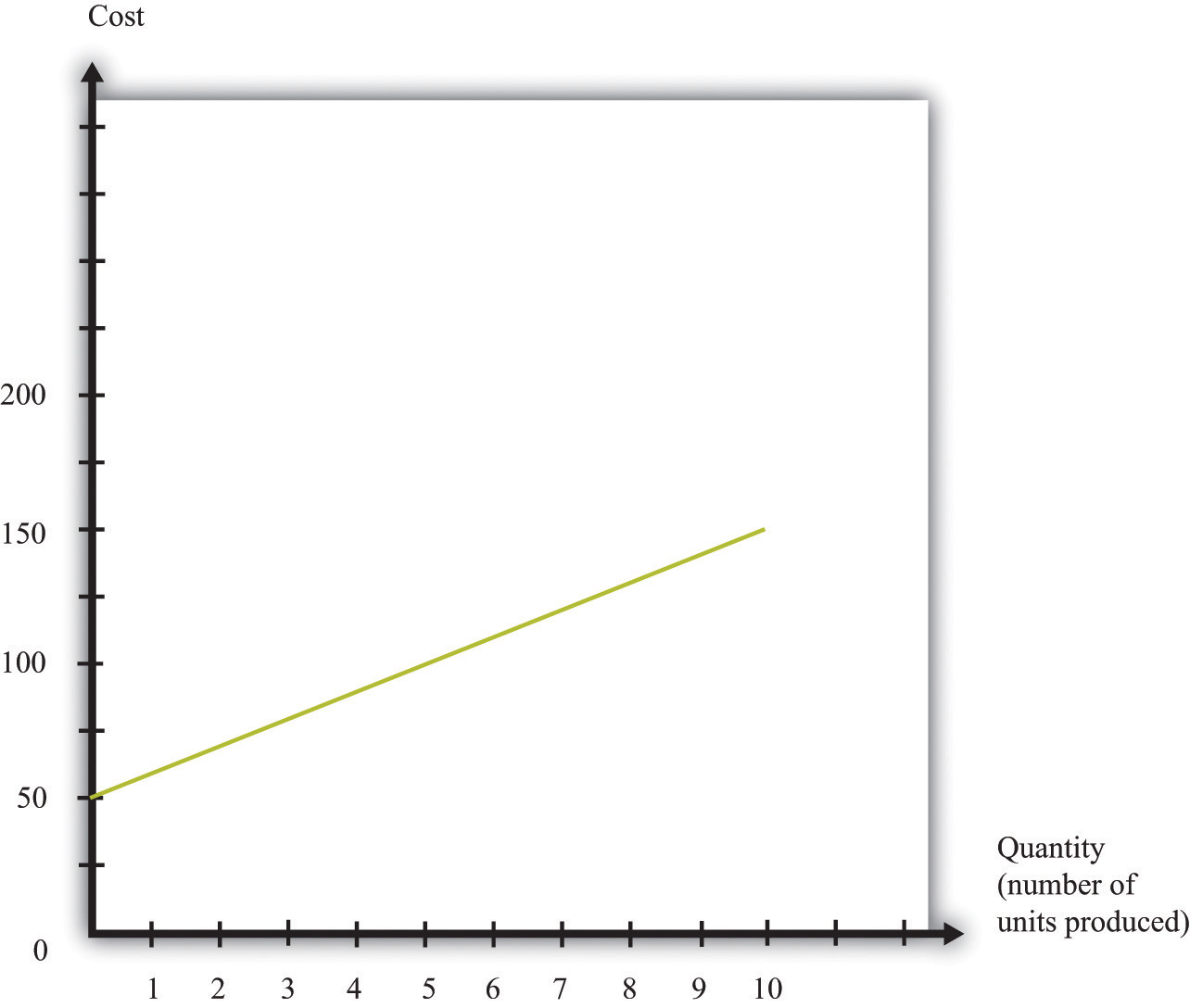## 6.3 The Costs of a Firm

### Learning Objective

1. What is marginal cost?
2. What costs matter for a firm’s pricing decision?

The goods and services that firms put up for sale don’t appear from nowhere. Firms produce these goods and incur costs as a result. When a marketing manager is thinking about the price that she sets, she must take into account that different prices lead to different levels of production and hence to different costs for the firm.

Your typical image of a firm probably involves a manufacturing process. This could be very simple indeed. For example, there are firms in Malaysia that produce palm oil. A firm in this context is little more than a big piece of machinery in the middle of a jungle of palm trees. The production process is hot, noisy, and very straightforward: (1) laborers harvest palm nuts from the trees surrounding the factory; (2) these palm nuts are crushed, heated, and pressed to extract the oil; and (3) the oil is placed into barrels and then sold. If you wanted to run a palm oil production business in Malaysia, you would need to purchase the following:

• Machine for extracting the oil
• Truck to transport the oil to market
• Generator to power the machinery
• Fuel to power the generator
• Gasoline for the truck
• Labor time from workers—to harvest the nuts, run the machinery, and transport the oil to market

That’s it. It is not difficult to become a palm oil entrepreneur! In this case, it is quite easy to list the main costs of production for the firm.

In other businesses, however, it is much more difficult. Imagine trying to make a similar list for Apple Computer, with all its different products, production plants in different countries, canteens for their workers, pension plans, and so on. Of course, Apple’s accountants still need to develop a list of Apple’s expenses, but they keep their jobs manageable by grouping Apple’s expenditures into various categories. If this were an accounting textbook, we would discuss these categories in detail. Our task here is simpler: we only need to determine how these costs matter for pricing decisions.

## Marginal Cost

Earlier, we showed how a firm’s revenues change when there is a change in quantity that the firm produces. If we also know how a firm’s costs change when there is a change in output, we have all the information we need for good pricing decisions. As with revenues, we scale this change by the size of the change in quantity. Figure 6.14 "Marginal Cost" shows how marginal costThe extra cost of producing an additional unit of output, which is equal to the change in cost divided by the change in quantity. fits into our road map for the chapter.

Marginal cost is the change in cost associated with a change in quantity of output produced:

Figure 6.14 Marginal CostWhen a firm sets a higher price, it sells a smaller quantity and its costs of production decrease.

Table 6.4 Marginal Cost

Output Total Costs Marginal Cost ($) 0 50 10 1 60 10 2 70 10 3 80 10 4 90 10 5 100 Table 6.4 "Marginal Cost" shows an example of a firm’s costs. It calculates marginal cost in the last column. We have presented this table with marginal cost on separate rows to emphasize that marginal cost is the cost of going from one level of output to the next. In our example, marginal cost—the cost of producing one more unit—is$10. If you want to produce one unit, it will cost you $60. If you want to produce two units, your must pay an additional$10 in costs, for a total of $70. If you want to produce three units, you must pay the$70 to produce the first two units, plus the additional marginal cost of $10, for a total cost of$80, and so on. Graphically, marginal cost is the slope of the cost line, as shown in Figure 6.15 "An Example of a Cost Function".

Figure 6.15 An Example of a Cost Function